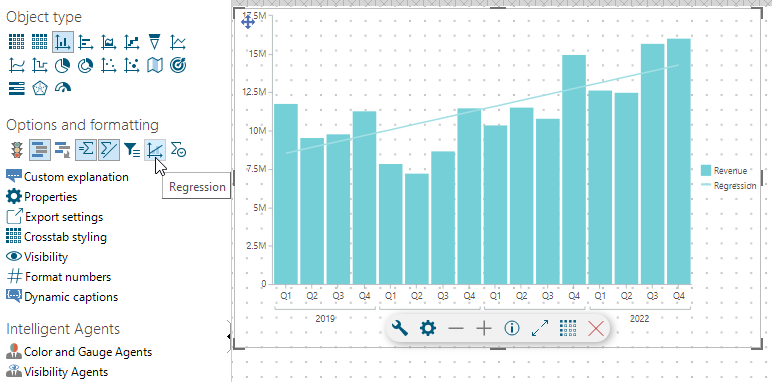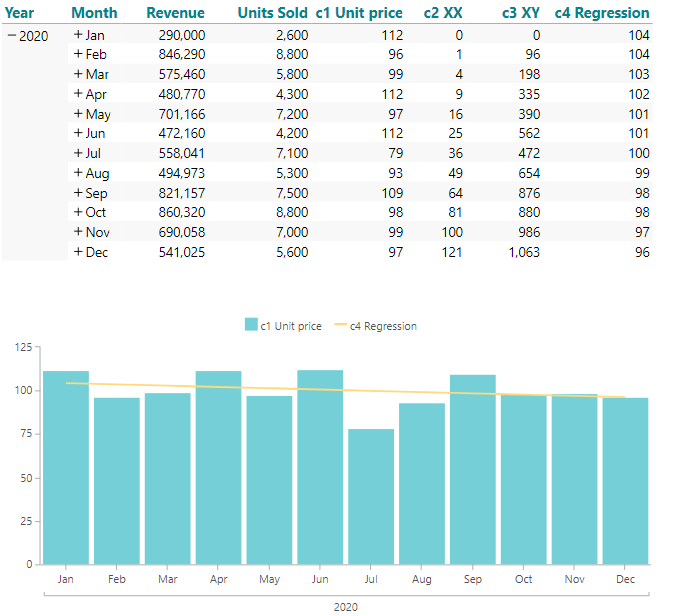# Custom Regression line

TARGIT comes with a built-in Regression line option:However, this built-in Regression line option only works on original measures from the data warehouse. If you add client based calculations to your object, the built-in Regression line will not work on those. Hence, you may want to calculate your own, custom Regression lines.

To do this, you will need to work with a total of three additional calculations to get to the desired (straight) regression line.

### Custom Regression line Example

In the following example, I am looking at a crosstab with two original measures: Revenue and Units Sold. From these I have calculated my 'c1 Unit price' as sum(d1, 0, m1) / sum(d1, 0, m2). The calculation is added as a calculated column and can be referred to as 'c1' in subsequent calculations.

Now I want to calculate regression values for my 'c1 Unit price' calculation - resulting in the regression line in the bar chart below.

To achieve the values for the custom regression, I will need to work with two intermediate calculated columns, 'c2 XX' and 'c3 XY' before getting to the final 'c4 Regression' calculation.The formulas you need, are these:

• For 'c2 XX' use:
• XX:((count(d1, d1:0, 0) - 1) * (count(d1, d1:0, 0) - 1))
• For 'c3 XY' use:
• XY:((count(d1, d1:0, 0) - 1) * sum(c1, 0, 0))
• For 'c4 Regression' use
• ((AvgY:(avg(c1, all, 0)) * AvgXX:(avg(c2, all, 0)) - (AvgX:((count(d1, all, 0) - 1) / 2) * AvgXY:(avg(c3, all, 0)))) / (AvgXX - AvgX * AvgX) + (count(d1, d1:0, 0) - 1) * (AvgXY - AvgX * AvgY) / (AvgXX - AvgX * AvgX))